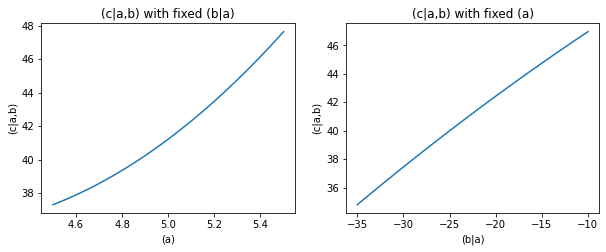# Influence Estimation#

:

%%capture
# execute the creation & training notebook first
%run "02-01-creation_and_training.ipynb"


After training we might want to know how much our parameters influence a certain target. We can do this with the .estimate_influences method.

Let’s start by estimating the influences on the parameter ‘(c|a,b)’.

:

causal_structure.estimate_influences(target='(c|a,b)')

:

(a)        0.675741
(b|a)      3.677125
(c|a,b)    1.000000
Name: influence on (c|a,b), dtype: float64


We see that ‘(c|a,b)’ influences itself by 1, that is to say it influences itself by 100%. ‘(a)’ influences it by 66%. This seems fairly in line with the R2-score in the performance evaluation section.

However, ‘(b|a)’ shows an influence above 100%. How can this be understood?

## Influences of correlated parameters#

The reason why ‘(b|a)’ shows an influence above 100% is that ‘(a)’ and ‘(b|a)’ are strongly anti-correlated

:

data.corr().loc[['(a)', '(b|a)'], ['(a)', '(b|a)']]

:

(a) (b|a)
(a) 1.000000 -0.959284
(b|a) -0.959284 1.000000

but they both have a positive effect on ‘(c|a,b)’.

:

test_data_a = np.linspace(4.5, 5.5, 100)
test_data_b = np.linspace(-35, -10, 100)

pl.figure(figsize=(10,3.5))
fig = pl.subplot(1,2,1)
fig.plot(test_data_a,
causal_structure.predict(data={'(a)': test_data_a, '(b|a)': -22.5})['(c|a,b)'],)
fig.set_title("(c|a,b) with fixed (b|a)")
fig.set_xlabel("(a)")
fig.set_ylabel("(c|a,b)")
fig = pl.subplot(1,2,2)
fig.plot(test_data_b,
causal_structure.predict(data={'(a)': 5., '(b|a)': test_data_b})['(c|a,b)'],)
fig.set_title("(c|a,b) with fixed (a)")
fig.set_xlabel("(b|a)")
fig.set_ylabel("(c|a,b)")

:

Text(0, 0.5, '(c|a,b)')So under normal circumstances the effects of ‘(a)’ and ‘(b|a)’ on ‘(c|a,b)’ cancel each other out to a large degree.

The influence estimator tells us what is the influence on the target, if you only change the parameter in question. Think of this as an intervention that breaks the strong correlation between ‘(a)’ and ‘(b|a)’.

This is why the influence of ‘(b|a)’ on ‘(c|a,b)’ is above 100%.

If we change the target of the influence estimation to ‘(b|a)’

:

causal_structure.estimate_influences(target='(b|a)')

:

(a)        0.919762
(b|a)      1.000000
(c|a,b)    0.000000
Name: influence on (b|a), dtype: float64


we see that ‘(c|a,b)’ has an influence of zero on ‘(b|a)’, even though you can predict ‘(b|a)’ from ‘(c|a,b)’ (see the “Backwards prediction” subsection in the prediction section). This is because estimate_influences respects causal directions. So effects do not influence causes.

For further details about the InfluenceEstimator see the corresponding section in the core-documentation.

In the next section we will have a look at rank estimation.